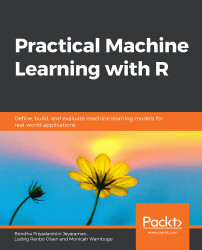•#### Practical Machine Learning with R#### Overview of this book

With huge amounts of data being generated every moment, businesses need applications that apply complex mathematical calculations to data repeatedly and at speed. With machine learning techniques and R, you can easily develop these kinds of applications in an efficient way. Practical Machine Learning with R begins by helping you grasp the basics of machine learning methods, while also highlighting how and why they work. You will understand how to get these algorithms to work in practice, rather than focusing on mathematical derivations. As you progress from one chapter to another, you will gain hands-on experience of building a machine learning solution in R. Next, using R packages such as rpart, random forest, and multiple imputation by chained equations (MICE), you will learn to implement algorithms including neural net classifier, decision trees, and linear and non-linear regression. As you progress through the book, you’ll delve into various machine learning techniques for both supervised and unsupervised learning approaches. In addition to this, you’ll gain insights into partitioning the datasets and mechanisms to evaluate the results from each model and be able to compare them. By the end of this book, you will have gained expertise in solving your business problems, starting by forming a good problem statement, selecting the most appropriate model to solve your problem, and then ensuring that you do not overtrain it.Free Chapter
An Introduction to Machine LearningData Cleaning and Pre-processingFeature EngineeringIntroduction to neuralnet and Evaluation MethodsLinear and Logistic Regression ModelsUnsupervised LearningAppendix## Summary

In this chapter, we learned about the machine learning process. The various steps are iterative in nature and ensure that the data is processed in a systematic manner. We explored the various evaluation metrics for evaluating a trained model. We also covered two types of variables: categorical and numeric.

We covered the different ways to view the summary of the data. We also delved into the various plots available in R for visualizing the data and for performing EDA. We looked at the German Credit, Boston Housing, and Diabetes datasets and performed some visualization on these datasets to understand them better. We also learned how to plot correlations for the features in the data and the ways to interpret them.

We looked into the common machine learning models used by data scientists. We also came to understand some of the types of models that can be used for numeric prediction and categorical prediction. Furthermore, we implemented a classifier in R and interpreted the results. We explored built-in datasets for building linear regression and classifier models.